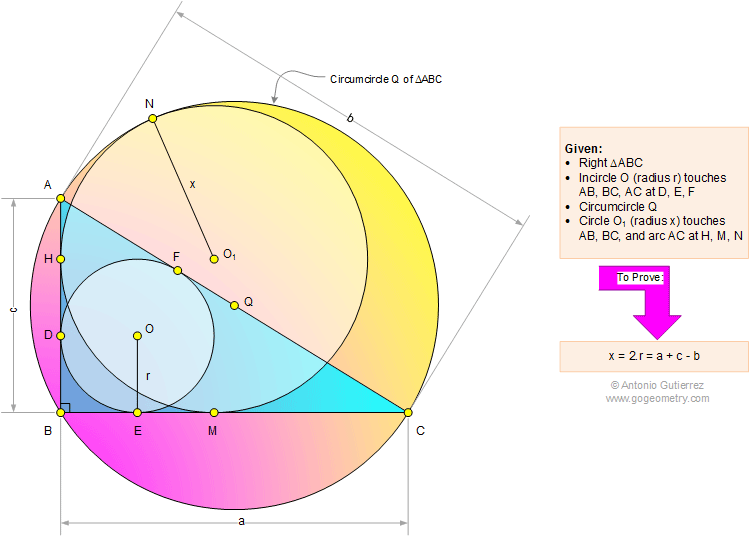Problem 1104: Right Triangle, Incircle, Circumcircle, Inscribed Circle, Radius, Tangent. Level: High School, SAT Prep, College Geometry

< PREVIOUS PROBLEM  |  NEXT PROBLEM >

 In a right triangle ABC (see the figure below) with circumcircle Q, the incircle O (radius r) is tangent to AB, BC, and AC at D, E, and F, respectively. Circle O1 (radius x) is tangent to AB, BC and arc AC at H, M, N, respectively. Prove that x = 2r = a + c - b.Home | Search | Geometry | Problems | All Problems | Open Problems | Visual Index | 1101-1110 | Triangle | Right Triangle | Circle | Incircle | Tangent Line | Circumcircle | Email | Post a comment or solutionLast updated: Mar 30, 2015, by Antonio Gutierrez